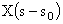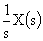LaPlace Transform Properties

In mathematics, the Laplace transform is one of the best known and most widely used integral transforms. It is commonly used to produce an easily solvable algebraic equation from an ordinary differential equation. It has many important applications in mathematics, physics, optics, electrical engineering, control engineering, signal processing, and probability theory.

In mathematics [and engineering], it is used for solving differential and integral equations. In physics and engineering, it is used for analysis of linear time-invariant systems such as electrical circuits, harmonic oscillators, optical devices, and mechanical systems. In this analysis, the Laplace transform is often interpreted as a transformation from the time-domain, in which inputs and outputs are functions of time, to the frequency-domain, where the same inputs and outputs are functions of complex angular frequency, in radians per unit time. Given a simple mathematical or functional description of an input or output to a system, the Laplace transform provides an alternative functional description that often simplifies the process of analyzing the behavior of the system, or in synthesizing a new system based on a set of specifications. - Wikipedia↔Signal LaPlace Transform↔↔↔↔↔(convolution)↔↔↔About RF CafeCopyright: 1996 - 2024Webmaster:    Kirt Blattenberger,    BSEE - KB3UON RF Cafe began life in 1996 as "RF Tools" in an AOL screen name web space totaling 2 MB. Its primary purpose was to provide me with ready access to commonly needed formulas and reference material while performing my work as an RF system and circuit design engineer. The Internet was still largely an unknown entity at the time and not much was available in the form of WYSIWYG ... All trademarks, copyrights, patents, and other rights of ownership to images and text used on the RF Cafe website are hereby acknowledged. My Hobby Website:  AirplanesAndRockets.com